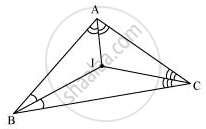Share

# In a Triangle Locate a Point in Its Interior Which is Equidistant from All the Sides of the Triangle. - CBSE Class 9 - Mathematics

ConceptInequalities in a Triangle

#### Question

In a triangle locate a point in its interior which is equidistant from all the sides of the triangle.

#### Solution

The point which is equidistant from all the sides of a triangle is called the incentre of the triangle. Incentre of a triangle is the intersection point of the angle bisectors of the interior angles of that triangle.Here, in ΔABC, we can find the incentre of this triangle by drawing the angle bisectors of the interior angles of this triangle. I is the point where these angle bisectors are intersecting each other. Therefore, I is the point equidistant from all the sides of ΔABC.

Is there an error in this question or solution?

#### APPEARS IN

NCERT Solution for Mathematics Class 9 (2018 to Current)
Chapter 7: Triangles
Ex. 7.50 | Q: 2 | Page no. 133

#### Video TutorialsVIEW ALL 

Solution In a Triangle Locate a Point in Its Interior Which is Equidistant from All the Sides of the Triangle. Concept: Inequalities in a Triangle.
S